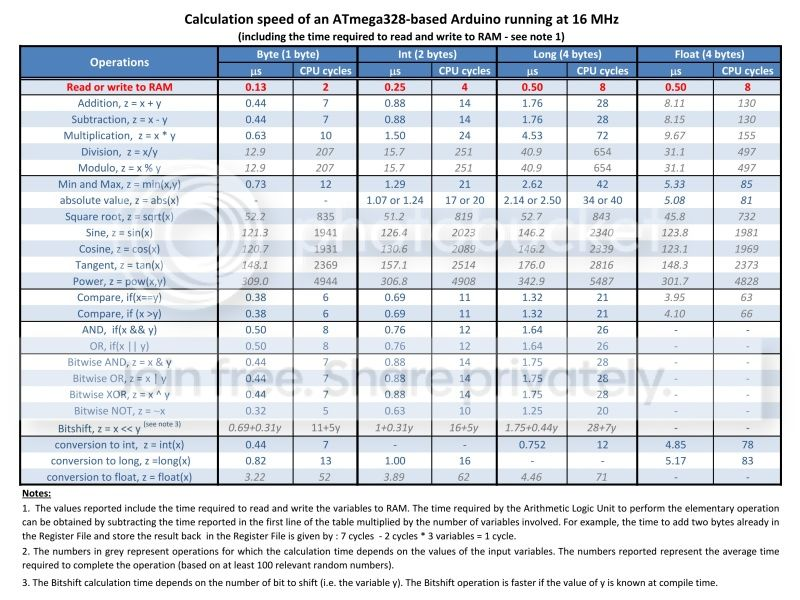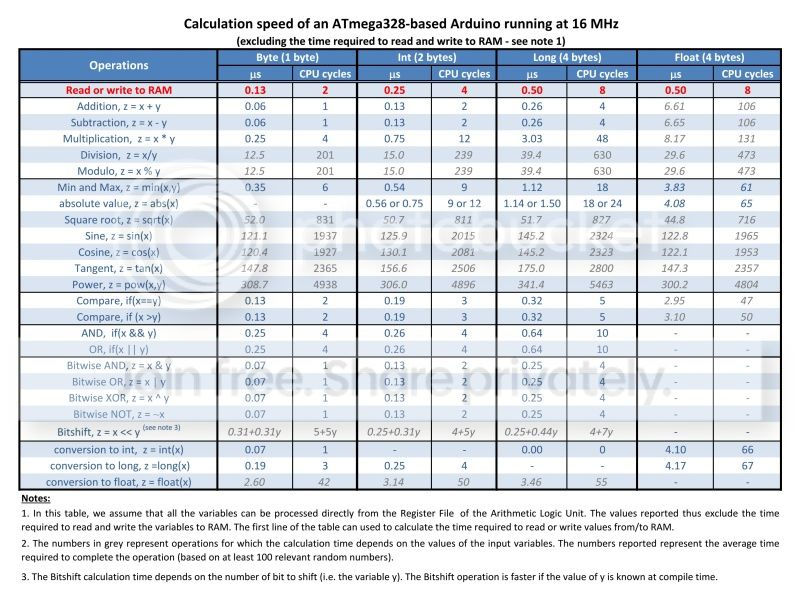Go Down

### Topic: Calculation speed tables (Read 8053 times)previous topic - next topic

#### DBgit##### Nov 23, 2013, 02:37 am
I had to work recently on a time sensitive project where I wanted to make many operations in less than 30 microseconds. Although some timing values can be found here and there in this forum, I thought that it could be useful to have a table showing the time taken to make some operations with an Arduino Uno (or other ATmega328-based Arduino running at 16 MHz). I thus tried to make my own measurements (see below for more details) and I ended up making somewhat detailed tables.

The first table shows the time taken to execute common Arduino commands. I also included few Assembly commands to show the potential speed gain for some operations. Also note that the time required for serial communications depends on the data rate and on whether the serial buffer is full or not.The second and third tables show the calculation speed of an ATmega328-based Arduino running at 16 MHz. It is important to note that the actual time taken to make an operation depends on whether the variables are already in Register File or if they must be first transferred from RAM. I thus decided to make two tables:

This table assumes that all the variables must be fetched from RAM (worst case scenario):This table assumes that all the variables are already available in the Register File (best case scenario):I also attached the tables in pdf format to this message.

Method used to make the tables:

Disclaimer: I have a limited experience with AVR programming and GCC compiler. During my tests, I found that measuring the time taken to run some commands is tricky because the compiler can perform optimizations that can alter timing measurements. It is also difficult to get rid of any overhead and measure precisely the time taken for each operation. So, let me know if you believe that the method I used for timing measurements is wrong for some reason.

Here is the code I used for the timing measurements:
Code: [Select]
`const int nb_of_interation_per_pass = 30000;const int nb_of_pass = 100;unsigned long initial_time = 0;unsigned long final_time = 0;float duration_dummy_loop = 0;float duration =0;float duration_sum = 0;// All variables used for calculations are declared globally and volatile to minimize// any possible compiler optimisation when performing the same operation multiple times. volatile int dummy = 0;volatile float float_1 = 0;volatile float float_2 = 0;volatile float float_3 = 0;volatile long long_1 = 0;volatile long long_2 = 0;volatile long long_3 = 0;volatile int int_1 = 0;volatile int int_2 = 0;volatile int int_3 = 0;volatile byte byte_1 = 0;volatile byte byte_2 = 0;volatile byte byte_3 = 0;volatile boolean bool_1 = 0;volatile boolean bool_2 = 0;volatile boolean bool_3 = 0;void setup() { Serial.begin (115200);}void loop() {    for (int j = 0; j < nb_of_pass ; j++) {             // STEP 1: We first calculate the time taken to run a dummy FOR loop to measure the overhead cause by the execution of the loop.       initial_time = micros();       for (int i = 0; i < nb_of_interation_per_pass ; i++) {        dummy++; // A dummy instruction is introduced here. If not, the compiler is smart enough to just skip the loop entirely...      }      final_time = micros();      duration_dummy_loop = float(final_time - initial_time)/nb_of_interation_per_pass; // The average duration of a dummy loop is calculated      dummy = 0;                   // STEP 2 (optional): Pick some relevant random numbers to test the command under random conditions. Make sure to pick numbers appropriate for your command (e.g. no negative number for the command "sqrt()")      randomSeed(micros()*analogRead(0));      byte_1 = random(0,256);      byte_2 = random(1,256);             // STEP 3: Calculation of the time taken to run the dummy FOR loop and the command to test.      initial_time = micros();      for (int i = 0; i < nb_of_interation_per_pass ; i++) {         dummy++; // The dummy instruction is also performed here so that we can remove the effect of the dummy FOR loop accurately.         // **************** PUT YOUR COMMAND TO TEST HERE ********************                  byte_3 = byte_1/byte_2; // Target command example         // **************** PUT YOUR COMMAND TO TEST HERE ********************      }      final_time = micros();            // STEP 4: Calculation of the time taken to run only the target command.      duration = float(final_time - initial_time)/nb_of_interation_per_pass - duration_dummy_loop;      duration_sum += duration;      dummy = 0;            Serial.print(j);      Serial.print(". ");      print_result(duration);      }    Serial.println();  Serial.println("********* FINAL AVERAGED VALUE *********** ");  print_result(duration_sum/nb_of_pass);  Serial.println("****************************************** ");  Serial.println();  duration_sum = 0;  delay(2000);}void print_result(float value_to_print){  Serial.print("Time to execute command: ");  Serial.print("\t");  Serial.print(value_to_print,3);  Serial.print(" us");  Serial.print("\t");  Serial.print(round(value_to_print*16));  Serial.println(" cycles");}`

The method I used can be summarized as follow:
1.   All variables are declared globally and volatile to minimize any possible compiler optimization.
2.   The time to run a dummy command a large number of times is measured using micros().
3.   The time to run the target command AND the dummy command the same number of times is measured using micros(). The time to run only the command is then obtained by a simple subtraction.

This technique should get rid of the overhead caused by the loop. The large number of iterations (I typically used 30000 iterations) also provides a relatively accurate timing despite the limited accuracy of micros(). After dividing by the number of iterations, the timing values can thus be measured to about ±0.02 us (~1/3 of a clock cycle). Also, I found that the timing of some operations depends on the values used to make the calculations (e.g. floating point operations, divisions, etc.). For these operations, I picked relevant random numbers and performed the timing calculation using at least 100 different values.

As all the variables are declared volatile, this code returns timing that includes the time required to read and write the variables to RAM. If we want only the time required by the Arithmetic Logic Unit to perform the operation, we have to subtract the time to read and write to RAM. The good news is that the time to access RAM can be measured easily. For example, writing to RAM corresponds to the time required to assign a known constant to a volatile variable (e.g. byte_1 = 128;).

From what I understand, the timing values I measured appear to be valid. For example, the measured times to access RAM (i.e. 2 clock cycles per byte) and to add two bytes (1 cycle if the bytes are in the Register) corresponds to that reported in the ATmega328 datasheet. Also, the time to execute __asm__("nop\n\t") is measured as 1 cycle as it should.#1
##### Nov 23, 2013, 02:43 am
How about things like SPI.transfer() vs shiftout() ?
Designing & building electrical circuits for over 25 years.  Screw Shield for Mega/Due/Uno,  Bobuino with ATMega1284P, & other '328P & '1284P creations & offerings at  my website.

#### DBgit#2
##### Nov 23, 2013, 02:47 am

How about things like SPI.transfer() vs shiftout() ?

The tables are indeed far from being complete... I'm ready to add more commands if there is an interest.

#### nickgammon#3
##### Nov 23, 2013, 03:12 am

My figures for digitalWriteFast for example:

Code: [Select]
`1. Time to execute command: 0.121 us 2 cycles`

That's a lot faster way of writing to a pin if you happen to know the pin number at compile time.
Please post technical questions on the forum, not by personal message. Thanks!

#### nickgammon#4
##### Nov 23, 2013, 03:14 am
You could try arithmetic with the "long long" type.Please post technical questions on the forum, not by personal message. Thanks!

#### DBgit#5
##### Nov 23, 2013, 03:24 am

Yes. Good idea. I did test those with my code, but I decided not to include them because they are not available by default in the Arduino environment. BTW, why digitalRead and digitalWrite are not replaced with their faster alternative? Is there any advantage of keeping the "normal" digitalRead and digitalWrite commands?

You could try arithmetic with the "long long" type.Why not! Although it might take a long long time to test all of the operations with this type!

#### retrolefty#6
##### Nov 23, 2013, 03:27 am

Yes. Good idea. I did test those with my code, but I decided not to include them because they are not available by default in the Arduino environment. BTW, why digitalRead and digitalWrite are not replaced with their faster alternative? Is there any advantage of keeping the "normal" digitalRead and digitalWrite commands?

You could try arithmetic with the "long long" type.Why not! Although it might take a long long time to test all of the operations with this type!

digitalRead and digitalWrite allow the pin number argument to be a variable, the fast version it must be a constant value. That's what accounts for most of the speed difference.

Lefty

#### DBgit#7
##### Nov 23, 2013, 03:37 am

digitalRead and digitalWrite allow the pin number argument to be a variable, the fast version it must be a constant value.

Hum... My understanding is that the fast version can also be used with a variable as the pin number. However, it will simply not speed up the code if the pin number is a variable or if the HIGH/LOW value is not known at compile time. So the fast version gives the same freedom as the normal version but can speed up dramatically the operation in some conditions.

#### nickgammon#8
##### Nov 23, 2013, 04:02 am
I think you are right but improvements to the core libraries can be a bit slow to be implemented.
Please post technical questions on the forum, not by personal message. Thanks!